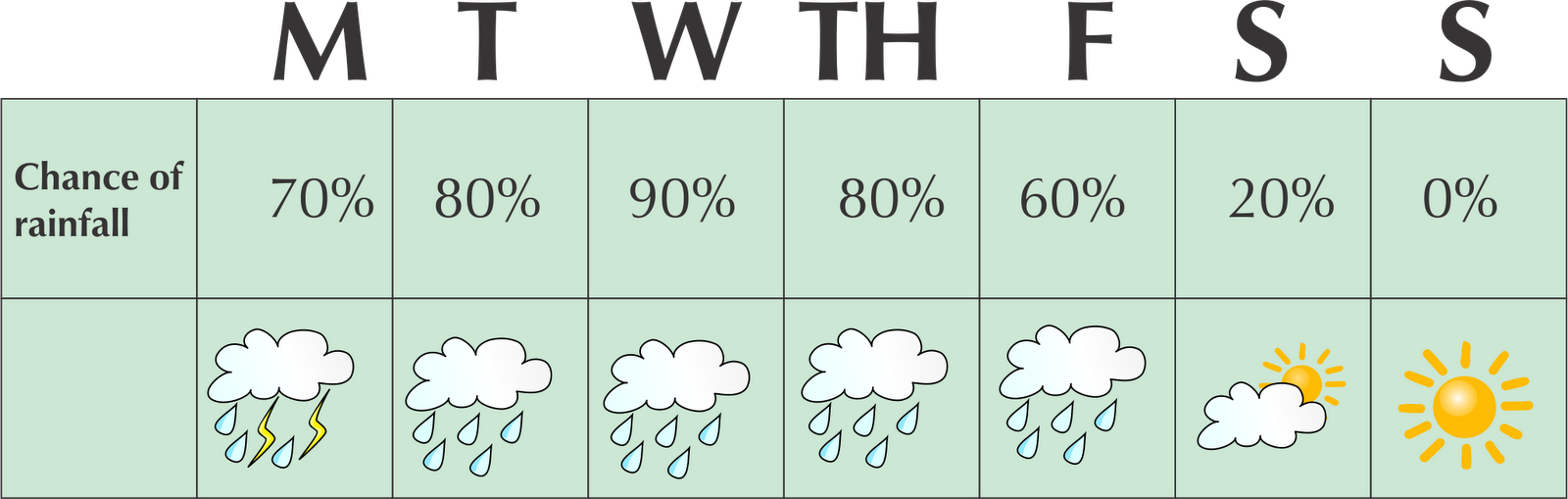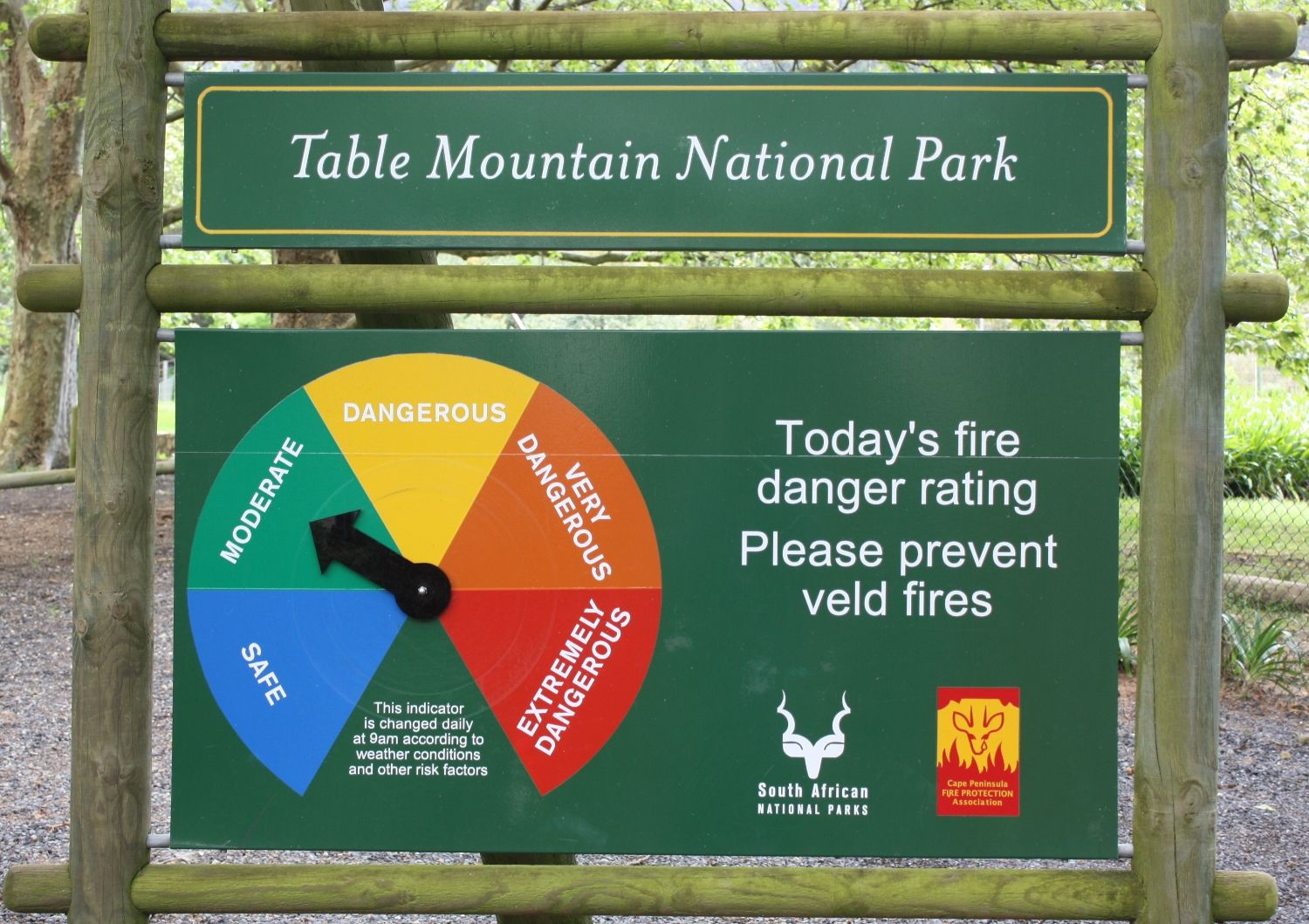Home Practice
For learners and parents For teachers and schools
Textbooks
Full catalogue
Pricing SupportLog in

We think you are located in United States. Is this correct?

# 7.5 Weather predictions

## 7.5 Weather predictions (EMG58)Have you ever wondered what it means when the weather report says that there is a $$\text{60}\%$$ chance of rain?

The $$\text{60}\%$$ chance is calculated in a similar way to how we have calculated probability in this chapter.

Forecasts like these are calculated by the South African Weather Service when they look at all other days in their database that have the same weather characteristics (temperature, pressure, humidity, etc.) and find it rained that on $$\text{60}\%$$ of days with the same weather characteristics.

Database
An organised collection of information, often collected over time that is organised in such a way that it is can be easily accessed, updated and managed. A database may take the form of paper, e.g. a telephone book is an example of a paper database. Facebook is an example of a computer database that stores friends' information and photos.

To calculate probability we divide the number of favourable outcomes by the total number of possible outcomes. If we want to know the probability that it will rain, this will be the number of days in our database that it rained divided by the total number of similar days in our database. If we have data for $$\text{100}$$ days with similar weather conditions (the sample space and therefore the denominator of our fraction), and on $$\text{60}$$ of these days it rained (a favourable outcome), the probability of rain on the next similar day is $$\frac{\text{60}}{\text{100}}$$ or $$\text{60}\%$$.

Since a $$\text{50}\%$$ probability means that an event has an equal chance of occurring and not occurring, $$\text{60}\%$$, which is greater than $$\text{50}\%$$, means that it is more likely to rain than not. But what is the probability that it won't rain? Remember that because the favourable outcomes represent all the possible ways that an event can occur, the total of the probabilities must be equal to $$\text{100}\%$$, so $$\text{100}\% - \text{60}\% = \text{40}\%$$, and so the probability that it will not rain is $$\text{40}\%$$.

## Working with weather predictions

Exercise 7.6

Try to find three different weather forecasts for your area. Listen to weather reports on the radio, watch them on the television and read them in newspapers. Collect information for a week about the predicted temperatures and the predicted rainfall.

Keep a record of what the weather is actually like on the day and note whether it was different or similar to the predicted weather.

If a weather prediction was very different from the weather that you experienced in reality, explain why this could have happened.

The prediction is based on past experience with similar weather conditions, and is not always correct.

Explain how weather forecasters use past data about the weather to predict the weather.

Weather forecasters look at the weather characteristics (temperature, pressure, humidity, etc.) and compare it to their accumulated data about weather patterns. Then they make a prediction based on this.

If a weather forecast says there is a $$\text{80}\%$$ chance that it will rain in your area, does that mean that you will definitely see rain? Is there a chance that it will not rain where you are? Explain.

Forecasters know that it rained on $$\text{80}\%$$ of the days in the past with similar weather conditions, but they can only state a probability, not exactly what will happen. There is also a $$\text{20}\%$$ probability of no rain.

## Weather predictions

Exercise 7.7

When one enters some games reserves and national parks in South Africa managed by SA National Parks you will find a sign in the form of a pie chart indicating the risk of a fire occurring in the park that particular day. Look at the picture of one of these fire risk pie charts and determine what weather predictions the fire risk probability is based upon.The fire risk probability depends on different aspects of the weather - primarily the probability of rain and high wind speeds. For example, if it has been very dry, and it is predicted that it will be windy, the risk of fire increases. If there is a $$\text{90}\%$$ chance of rain predicted, the risk if fire is reduced.